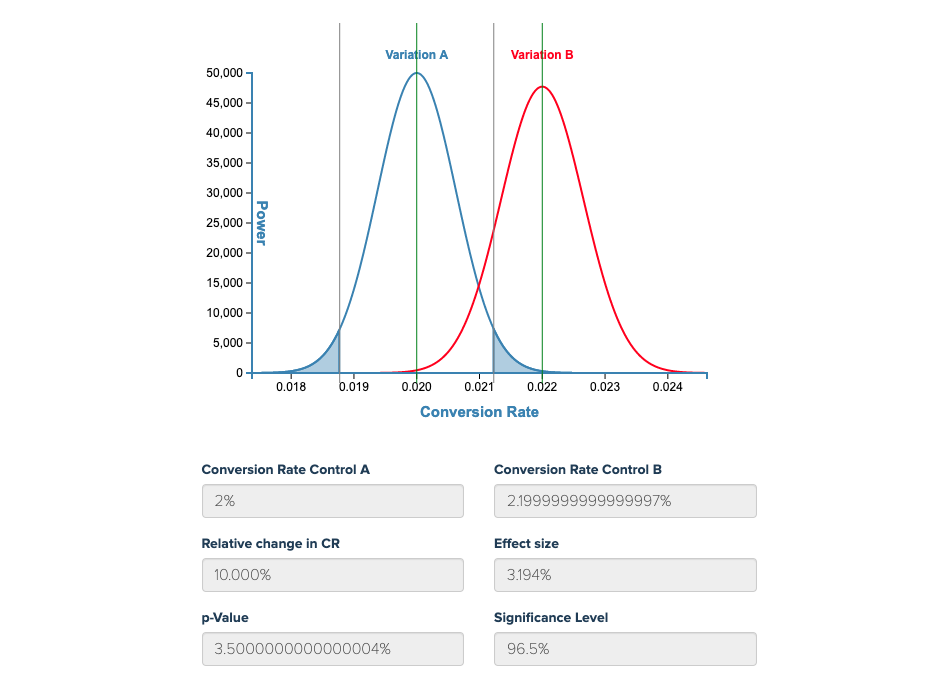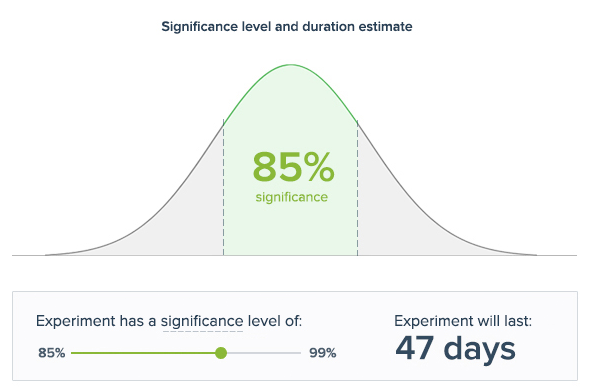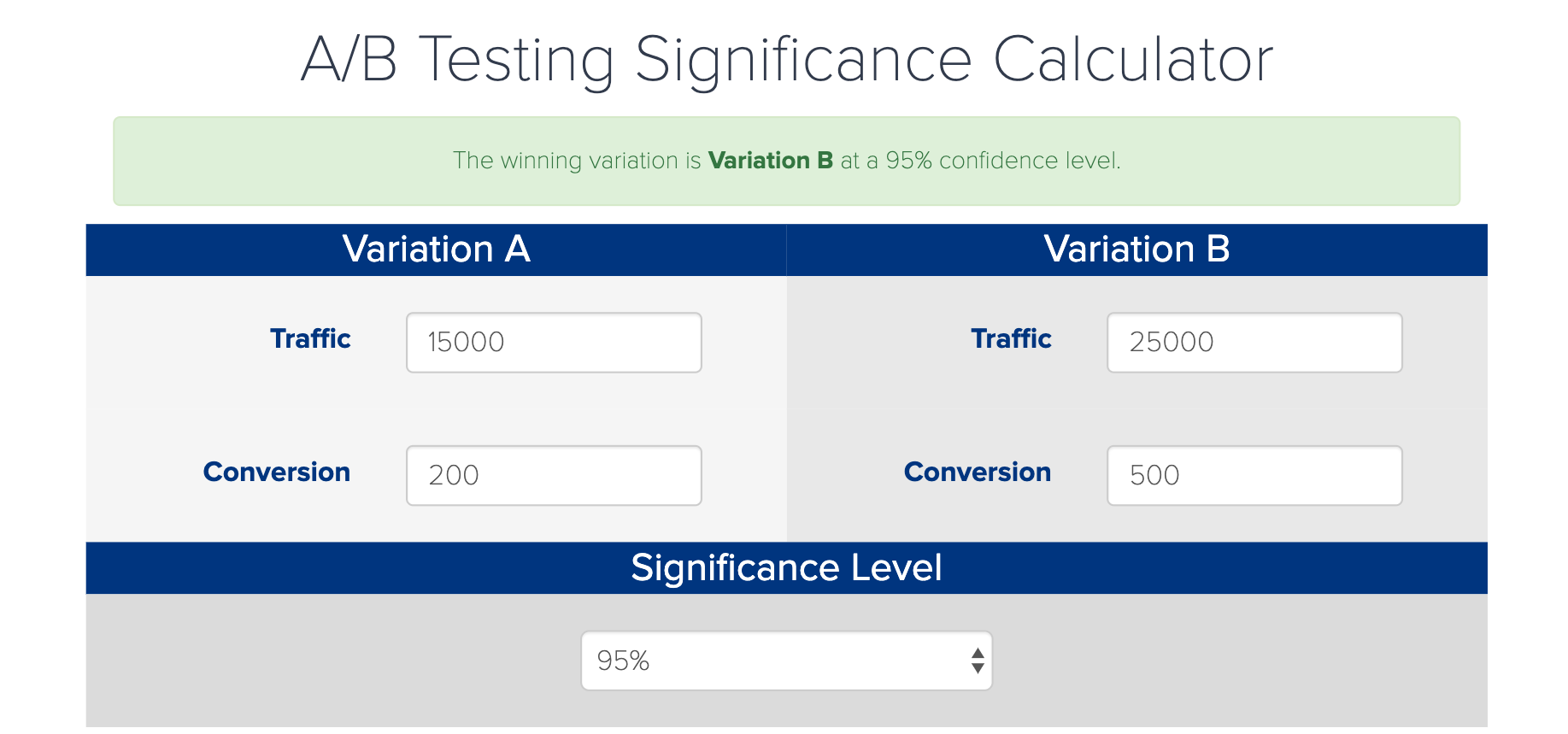# AB test significance: The minimum sample needed for reliable results

To get reliable results from your A/B test, you need statistically significant data. Gathering enough data to produce statistical insights is the main reason why most tests run for longer than expected. AB test significance is achieved when you believe your results are NOT the result of random chance. So, a test that has achieved statistical significance at a 95% CONFIDENCE LEVEL would occur through chance 5% of the time (1 in every 20 similar experiments).## The Minimum Sample Size Required For AB Test Significance

To calculate the sample size required for your test, you need to know the CONVERSION RATE for your original page and the EFFECT SIZE of your new scenario. You also need to set a confidence level that will be used to determine if your results are significant.

Your effect size is the magnitude of the difference produced by your new scenario. In website optimization this is usually your conversion rate UPLIFT (the percentage increase in your conversion rate).

With a higher original conversion rate, your effect size (the uplift caused by your experiment) will be larger. An uplift of 25% is much greater if your original conversion rate is 16% (Scenario 1) than it is if your original conversion rate is 4% (Scenario 2). Your new conversion rates would be…

• Scenario 1: Version A – 16% / Version B – 20%
• Scenario 2: Version A – 4% / Version B – 5%

If you knew in advance what the effect size of your experiment would be, you could calculate the sample size required to achieve AB test significance. However, in most cases, the effect size is unknown until midway through the test. That’s why Convertize predicts the days required your experiment to achieve significance at the same time as calculating the effect size of your test.These are some A/B testing scenarios, based on common parameters…

• CR 3%     Uplift 15%   Confidence Level 95%    Minimum Sample Size = 19056
• CR 10%   Uplift 15%   Confidence Level 95%    Minimum Sample Size = 5272
• CR 10%   Uplift 5%     Confidence Level 95%    Minimum Sample Size = 45499

For a step-by-step guide to AB test significance and sample sizes, read this Introduction to A/B testing.

## Calculating Your A/B Test Significance

If you have performed an A/B test and you want to calculate the significance of the results yourself, you can use this simple AB testing calculator. All you need to know is the number of visitors your pages have received and the number of conversions they have produced. The calculator assumes a confidence level of 95% (as this is the industry standard).